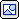TI 主页 >  半导体 >   设计资源 >  分压器确定一组分压电阻China (中文内容)

# 分压器确定一组分压电阻 VOLT-DIVIDER-CALC 此产品已上市，且可供购买。 可提供某些产品的较新替代品。

## VOLT-DIVIDER-CALC:Voltage Divider Determines A Set of Resistors for a Voltage Divider

ACTIVE

##### 描述VOLT-DIVIDER-CALC quickly determines a set of resistors for a voltage divider. This KnowledgeBase Javascript utility can be used to find a set of resistors for a voltage divider to achieve the desired output voltage. This calculator can also be used to design non-inverting attentuation circuits.

Initiate VOLT-DIVIDER-CALC